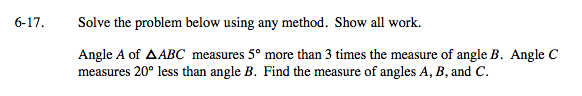### Home > GC > Chapter 6 > Lesson 6.1.2 > Problem6-17

6-17.Set up equations that represent the information you are given.

What is the sum of the angles within a triangle?
How can you use this to find ∠B?

One you know m∠B, solve for angles A and C.

m∠A = 3m∠B + 5°

m∠C = m∠B − 20°

m∠A + m∠B + m∠C = 180°

Substitute.
(3m∠B + 5°) + m∠B + (m∠B − 20°) = 180°

Solve for B.